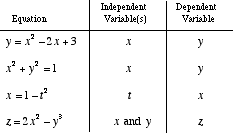index: click on a letter A B C D E F G H I J K L M N O P Q R S T U V W X Y Z A to Z index index: subject areas numbers & symbols sets, logic, proofs geometry algebra trigonometry advanced algebra & pre-calculus calculus advanced topics probability & statistics real world applications multimedia entrieswww.mathwords.com about mathwords website feedback

 Independent Variable A variable in an equation that may have its value freely chosen without considering values of any other variable. For equations such as y = 3x – 2, the independent variable is x. The variable y is not independent since it depends on the number chosen for x. Formally, an independent variable is a variable which can be assigned any permissible value without any restriction imposed by any other variable.See also Dependent variable